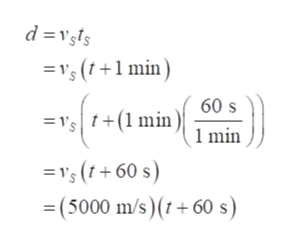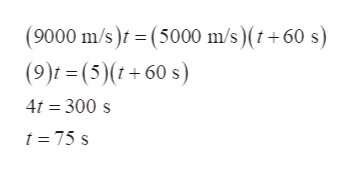# An earthquake emits both S-waves and P-waves which travel at different speeds through the Earth. A P-wave travels at 9 000 m/s and an S-wave travels at 5 000 m/s. If P-waves are received at a seismic station 1.00 minute before an S-wave arrives, how far away is the earthquake center?

Question

An earthquake emits both S-waves and P-waves which travel at different speeds through the Earth. A P-wave travels at 9 000 m/s and an S-wave travels at 5 000 m/s. If P-waves are received at a seismic station 1.00 minute before an S-wave arrives, how far away is the earthquake center?
check_circle

Step 1

Consider d be the distance between the station and the earthquake centre. Also, consider the time taken by the P waves to reach the station be t.

Therefore,

The distance d can be expressed as:

Using P waves:

Step 2

Also, using S waves: The S wave reaches 1 min late. Therefore,help_outlineImage Transcriptionclosedvsis = ' (1+1 min 60 s ="s | t+(1 min )\ 1 min = 's (1+60 s) = (5000 m/s)(t+60 s fullscreen
Step 3

Equate the distance obtained fr...help_outlineImage Transcriptionclose(9000 m/s) = (5000 m/s) (t+60 s) (9)f=(5)(t+60 s) 4t 300 s t=75 s fullscreen

### Want to see the full answer?

See Solution

#### Want to see this answer and more?

Solutions are written by subject experts who are available 24/7. Questions are typically answered within 1 hour.*

See Solution
*Response times may vary by subject and question.
Tagged in

### Physics